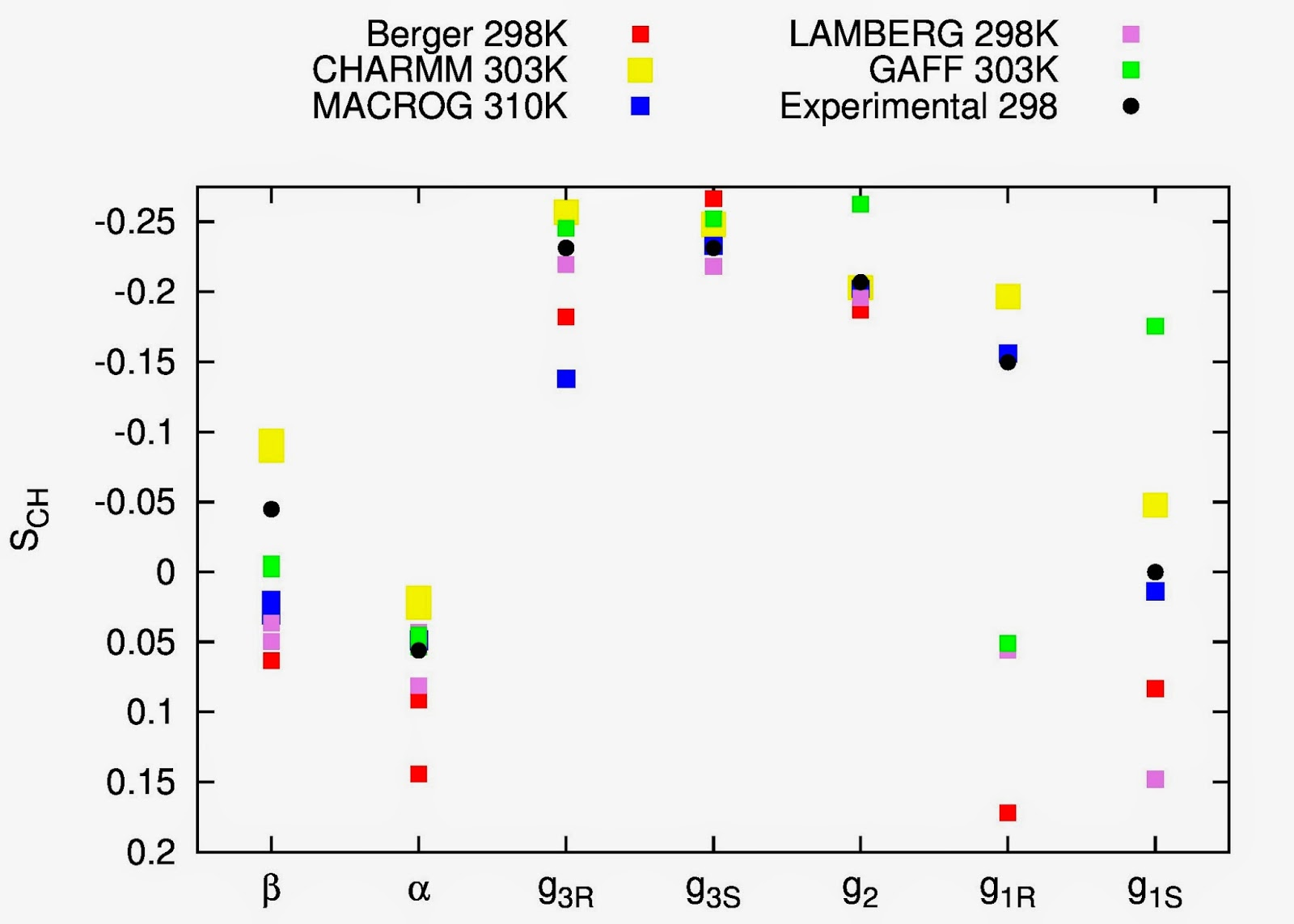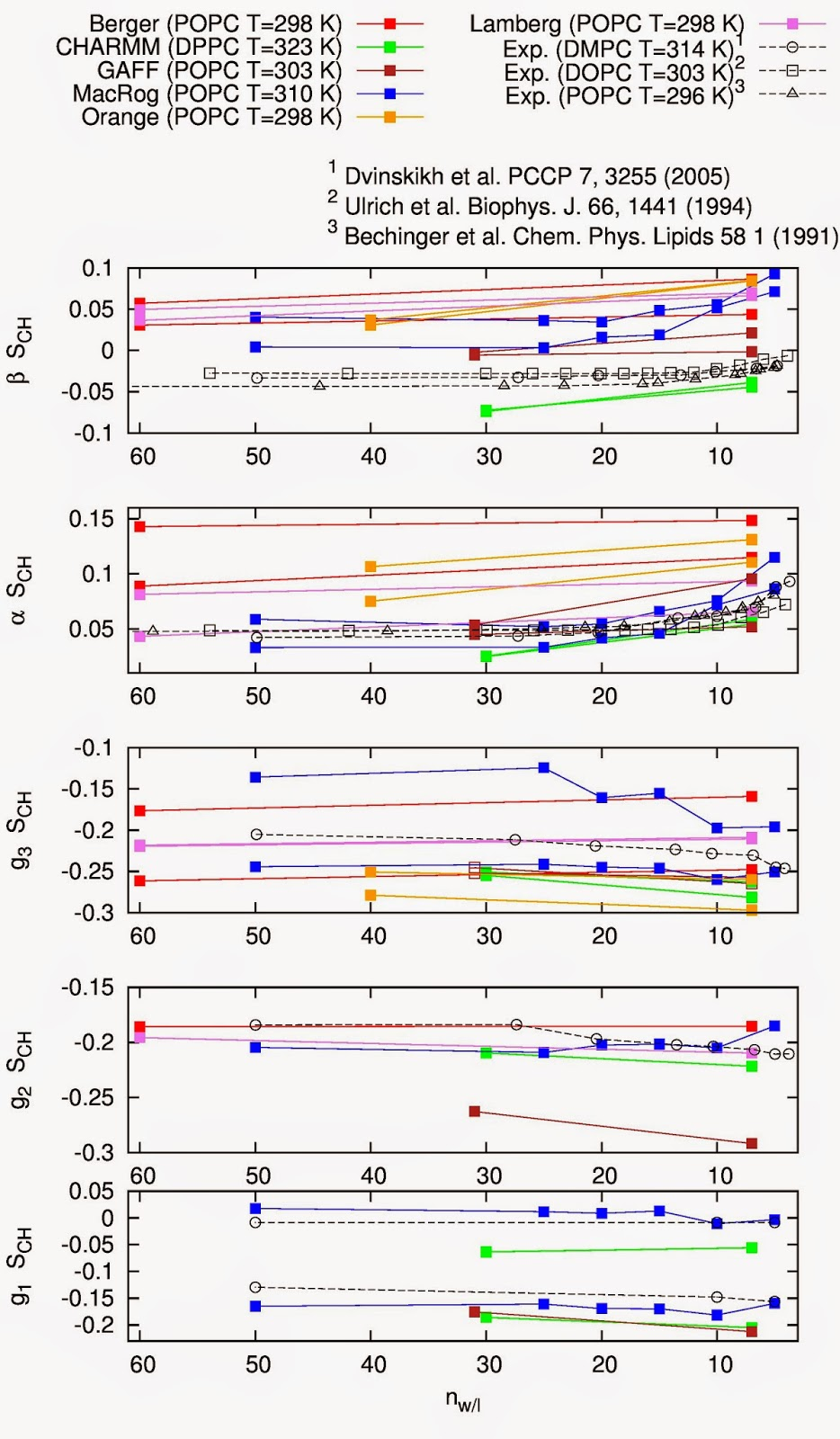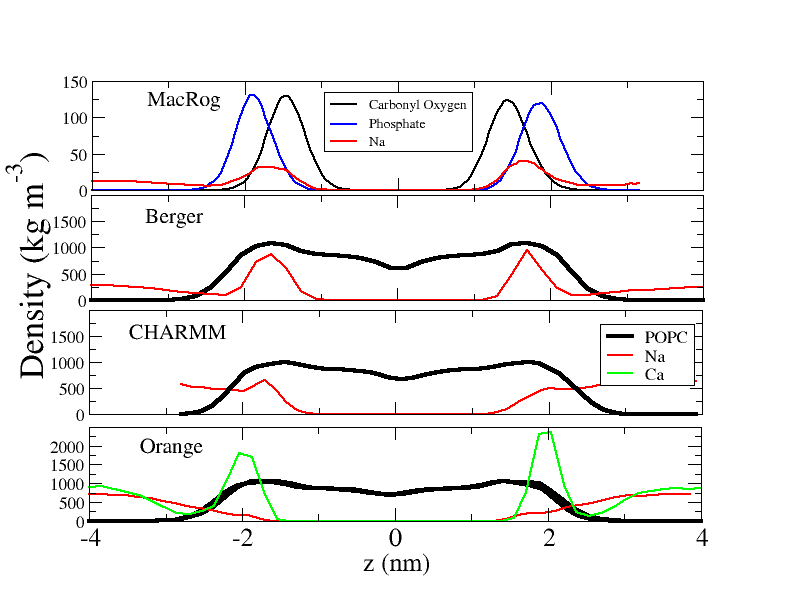## Monday, May 19, 2014

### Towards a new version of the manuscript

In the opening post of the nmrlipids project, published simultaneously with the accompanying manuscript, it was written that:
"The ultimate goal of this blog is to find an atomistic (preferably united-atom) force field that reproduces the experimental properties discussed in the manuscript. Naturally the optimal situation would be that some of the already available force fields would fulfill this goal. If this, however, turns out not to be the case, the goal will be to find the appropriate modifications."
As discussed in The lipid forcefield comparison post, none of the tested united-atom force fields fulfills the goal$$^1$$. The same post concluded that three all-atom force fields (CHARMM36, GAFFlipids and MacRog) gave a reasonable agreement with experiments for a fully hydrated pure bilayer, when the absolute values of headgroup and glycerol order parameters were compared. Since then, the comparison has been refined to include the sign of the order parameters as well as the R/S labeling of hydrogens. Fig. 1 shows an up to date comparison of the top three all-atom force fields, the Berger force field, and the Lamberg parameters (currently in development in this blog) to experiments.Figure 1. A set of simulation results for the POPC headgroup and glycerol order parameters reported in the blog is compared to experiments.

With these refinements (sign and R/S labeling) it becomes clear that the order parameters for the g$$_1$$ group in the GAFFlipid force field are not in a reasonable  agreement with experiments. Thus there are only two all-atom models left to consider: CHARMM36 and MacRog. Interestingly, as discussed in the post Response of headgroup and glycerol order parameters to changing conditions: Results, the responses of these two force fields to changing conditions (dehydration, ion concentration and cholesterol content) are in qualitative agreement with experiments. However, the Na$$^+$$ association with the bilayer is clearly too strong for MacRog; the association is less for CHARMM36, but more simulations are needed to complete the comparison to experiments.

In conclusion, CHARMM36 is a force field that relatively well satisfies the original goal of the nmrlipids project. In this respect, we could now just write up the manuscript, submit and finish the project.

Having said that, I still think that it would be very useful to have also a united-atom model that fulfills the original goal. Regarding the studies of pure lipid bilayers, CHARMM36 appears significantly slower than united-atom models and also slower than other all-atom models. Based on my personal experience (I have not investigated it further) I think the slowness is due to the Lennard-Jones interactions of the water hydrogens in the CHARMM water model$$^2$$. In addition to speed, there will probably be interest in using a lipid model with correct molecular structure in combination with proteins (or other molecules) that lack a model combatible with the CHARMM36 lipids.

If we manage to get correct structures for one united atom model, the same procedure could probably be used for other models as well. A promising method to tune the potentials to get the correct order parameters was already reported in this blog by Antti Lamberg in March. However, at the time, we had not considered the signs and the R/S labeling and thus the order parameters were matched to wrong values.

On the basis of these perpectives, I suggest that we make one further attempt to correct the order parameters for the Berger model in the following way:
1. We calculate the dihedral distributions from the CHARMM36 (and MacRog) for the glycerol and headgroup region.
2. We set up dihedral potentials for the Berger model such that they resemble the ones in the CHARMM36 (and/or MacRog).
3. We use these potentials as a starting point for a Lamberg-style optimization.
I think that this procedure has potential to produce a united-atom model that will have the correct glycerol and headgroup structures. If this procedure does not succeed, I suggest we finish this project and publish the results; further attempts to fix united-atom model can be continued in another project in any case. I will already start to prepare the next version of the manuscript.

First results for step 1 of the procedure. As preliminary work for the above procedure I plotted 40 consecutive lipid structures observed in Berger and CHARMM36 simulations such that the glycerol groups were aligned. The results are shown in Figs. 2 and 3.Figure 2. 40 consecutive ($$\Delta$$t=1.25 ns) structures observed in a Berger simulations (POPC, T=298K). Hydrogens have been added to the visualization by the Gromacs protonate program in the same way as for the order parameter calculation.Figure 3. 40 consecutive ($$\Delta$$t=1.25 ns) structures observed in a CHARMM36 simulation (POPC, T=303K).
Comparing the Figs. 2 and 3 one can clearly see that the conformations sampled by the g$$_1$$ and g$$_3$$ groups significantly differ between the models - which is no surprise as the models predict different order parameters. In all configurations of the CHARMM36 model the hydrogens of the g$$_1$$ group are pointing to the same direction, indicating a rather rigid dihedral in the g$$_1$$-g$$_2$$ bond. In the Berger model, the g$$_1$$-g$$_2$$ bond seems to be significantly more flexible. The situation is opposite for the g$$_3$$ group: the hydrogens are pointing in the same direction in almost all configurations for the Berger model, while CHARMM36 seems to be significantly more flexible. This difference seems to have significant effect to the conformations of the whole PC headgroup. (When looking at the figures, it should be noted that the molecules are shown from different angles to make the above points more clear.)

I am currently processing some ideas how to proceed to the step 2 in the procedure. I will report the progress in the near future. It would be also interesting to see structural figures with aligned glycerol from the MacRog model.

$$^1$$The recent united atom CHARMM has not been tested yet. The authors of the model state that "The headgroup is still an AA potential in C36-UA, so only the chain SCDs were compared." Thus similar results than in CHARMM36 are expected. However, the united atom CHARMM might still be quite slow due to the water model. Some results with the model would be interesting.

$$^2$$Using regular TIP3P model does not seem to be a solution since significant changes in phase behaviour was reported by Piggot et al.

## Friday, May 2, 2014

### Response of headgroup and glycerol order parameters to changing conditions: Results

During our project, the responses of headgroup and glycerol order parameters to hydration level, ion concentration and cholesterol content have already been reported for several models, and the results have been discussed to some extent in various comments. This post reviews the results for the responses reported in the nmrlipids-project so far.

Note on signs.
In the original manuscript and in the plots previously published in the blog (dehydration, NaCl, CaCl), the responses of the order parameters were discussed only in terms of their absolute values. From these plots the response of the $$\beta$$-carbon order parameter in all force fields except in CHARMM seemed to be qualitatively different than in experiments. Recently we have started to consider also the signs of the order parameters in addition to their magnitude. Even though the signs are not explicitly measured when responses are considered experimentally, it is reasonable to assume that the signs are not changing except for the case of adding a large amount of charges to the bilayer. Throughout this posting the signs of the order parameters are taken into account. For dehydration and ions the signs are relevant for the conclusions

The order parameters as a function of hydration level. It is clear from Fig. 1 that in experiments dehydration leads to more positive order parameters for the $$\beta$$ and $$\alpha$$ carbons. Results from all the experiments are in general very similar, despite some variation in lipid compositions and temperatures. The glycerol order parameters have been measured only in one experiment, where a decrease with dehydration was observed for the g$$_3$$ and g$$_2$$, while g$$_1$$ remains unchanged.Figure 1. Order parameters as a function of hydration level from the simulations reported through this blog and from the experiments by Dvinskikh et al. (2005), Ulrich et al. (1994) and Bechinger et al. (1991) In these experiments only absolute values were measured. The signs are assumed to be the same as in fully hydrated conditions measured by Hong et al. (1995), Hong et al. (1995) and Gross et al. (1997). Simulation values for some carbon segments are not seen since they are beyond the scale of the y-axis.

In simulations, as in experiments, the $$\beta$$- and $$\alpha$$-carbon order parameters increase with dehydration - even though the magnitudes are significantly different between different models and some models show significant forking. Note that this qualitative agreement between simulations and experiments for the $$\beta$$-carbon dehydration response differs from the conclusions of the original manuscript and of the discussions in the nmrlipids-blog. The reason for this is that previously only the absolute values have been discussed and many many force fields give wrong sign for the $$\beta$$-carbon order parameter: Increase in a negative order parameter decreases its absolute value (as seen in the experiments) while increase in a positive order parameter increases its absolute value (as seen in most simulations).

When the comparison between simulations and experiments is extended to the glycerol region, only the CHARMM36 force field can be interpreted to have all the order parameters quantitatively close to experiments, and to have qualitatively correct response to dehydration. However, the changes in the order parameters and difference between MacRog results and experiments are relatively small in the glycerol region.

The order parameters as a function of NaCl or CaCl$$_2$$ concentration. Fig. 2 shows the effect of NaCl on headgroup and glycerol order parameters.Figure 2. Order parameters as a function of NaCl concentration from simulations reported in the blog and from experiments by Akutsu et al. (1981). The signs are assumed to be the same as measured by Hong et al. (1995), Hong et al. (1995) and Gross et al. (1997). The effect of ions to the g$$_2$$ and g$$_3$$ were not measured, thus only the values without ions are shown. The straight line between the results with and without ions is plotted to guide the eye. The results with ions for the GAFFlipids are not available. The results for the Lamberg model are not included since the model is still under development.

The effect of penetrating charges on lipid bilayer properties has often been studied with NMR by measuring the $$\beta$$ and $$\alpha$$ order parameters [Seelig et al. (1987), Semchyschyn et al. (2004) and references therein]. For this reason let us take a closer look of these order parameters as a function of NaCl and CaCl$$_2$$ (Fig. 3).Figure 3. Order parameters for $$\beta$$ and $$\alpha$$ carbons as a function of NaCl (left column) and CaCl$$_2$$ (right column) concentrations from simulations reported in the blog and from the experiments by Akutsu et al. (1981). The Berger results are shown in the plot even though some of their values are beyond the y-axis scale.

It is clear from Figs. 2 and 3 that NaCl induces a dramatic decrease of the $$\beta$$- and $$\alpha$$-carbon order parameters in Berger and MacRog models; the effect is too strong compared to the experiments. Qualitatively, however, this does correspond to the experimentally observed effect of penetrating charges (multivalent ions, anionic surfactants) into PC bilayers [Seelig et al. (1987)]. This qualitative correspondence was hidden in our previous considerations (NaCl, CaCl) in which only the absolute values were compared (similarly to the dehydration case, discussed above). The most obvious explanation for this finding is that the Na$$^+$$-partitioning into the bilayer is too strong in Berger and MacRog models.

The situation is different in CHARMM36 and Orange force fields: Adding NaCl induces small or nonexistent changes at the $$\beta$$ and $$\alpha$$ order parameters. This signals significantly lower partitioning.  The data as a function of CaCl$$_2$$ with Orange force field (Fig. 4), however, shows a disctinct difference to the Na$$^+$$ case: Ca$$^{2+}$$ significantly reduces the $$\beta$$- and $$\alpha$$-order parameters. This disctinction has been seen also in experiments, where it has been interpreted as a signature of the different in partitioning of Na$$^{+}$$ and Ca$$^{2+}$$ into PC bilayers. (Note, however, that in the Orange force field addition of Ca$$^{2+}$$ leads to changes in order parameters that are larger than in experiments.)

To check the validity of interpreting the order parameter results in terms of differences partitioning affinities, Fig. 4 shows the density profiles for lipids and ions in different simulations.Figure 4. Density profiles for lipids and ions from different simulations reported in the nmrlipids-blog. The ion densitied are multiplied with 4 in MacRog, and with 50 in other models to make them visible with the used y-axis scale.
By comparing Figs 2, 3 and 4 it becomes clear that Na$$^+$$ binding is stronger (Fig. 4) in the force fields in which the Na$$^+$$-induced changes in the order parameters are larger (Figs. 2 and 3), i.e., in Berger and MacRog. In CHARMM36 and Orange, in which the Na$$^+$$-induced changes in order parameters are smaller, also the partitioning of Na$$^+$$ is significantly less. It seems that the correlation between ion binding and order parameter changes that was suggested from the NMR experiments [Akutsu et al. (1981), Seelig et al. (1987)] can be reproduced in MD simulations. This is an important finding. However, to conclude how straightforward the connection between order parameters and ion partitioning is, and if the binding affinity in the Orange and CHARMM36 force fields is in agreement with experiments, we have to make more quantitative analysis on binding and run simulations with different ion concentrations.

As a final note in ions, it is interesting that the Berger and Orange simulations used the same ion model. Thus, the Na$$^+$$ partitioning can be largely reduced just by modifying the lipid parameters. More detailed analysis of the relevant changes, however, is difficult since the Orange parameters are not yet publicly available.

The order parameters as function of cholesterol concentration. Based on the reported data, both force fields (MacRog and CHARMM36) reproduce the response to cholesterol relatively well (Fig. 5). This was not the case for the Berger, as discussed in the original manuscript. However, for MacRog high cholesterol contents have not been reported and it is still possible for discrepancies to occur there.Figure 5. Order parameters as a function of cholesterol concentration from simulations reported in the blog and from experiments by Ferreira et al. (signs taken from Hong et al. (1995), Hong et al. (1995) and Gross et al. (1997) and assumed to be unchanged with cholesterol concentration). In the CHARMM36 results the cholesterol force field by Lim et al. (2012) was used.The results from Berger force field are shown even though some values are beyond the y-axis scale. For other force fields the data is not available.
Preliminary conclusions
• When the signs are taken into account, the qualitative response to dehydration and penetrating charges seems to agree with experiments in all tested force fields.
• As suggested in experiments, the changes in headgroup order parameters can be related to the amount on penetrated charge also in simulations.
• Comparison to experiments shows Na$$^+$$ partitioning to be too strong in Berger and MacRog models.
• More detailed studies are needed to conclude if Na$$^+$$ partitioning in CHARMM36 and Orange agrees with experiments.
• Response to cholesterol seems to be relative realistic in CHARMM36 and MacRog.